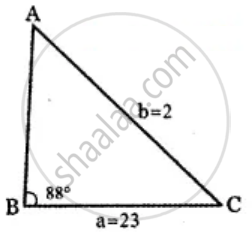Tamil Nadu Board of Secondary EducationHSC Arts Class 11th

# Determine whether the following measurements produce one triangle, two triangles or no triangle:∠B = 88°, a = 23, b = 2. Solve if solution exists - Mathematics

Sum

Determine whether the following measurements produce one triangle, two triangles or no triangle:
∠B = 88°, a = 23, b = 2. Solve if solution exists

#### SolutionUsing sine formula

"a"/sin"A" = "b"/sin"B"

sin"A"/"a" = sin"B"/"b"

sin"A"/23 = (sin88^circ)/2

sin A = (23 xx sin 88^circ)/2

= 23 xx (1.998)/2

= 23 × 0.999

= 22.99

Which is not possible

∴ Solution of the given triangle does not exsit.

Concept: Application to Triangle
Is there an error in this question or solution?

Share